## Static Electricity Review

#### Navigate to:

Review Session Home - Topic Listing

Static Electricity - Home || Printable Version || Questions with Links

Answers to Questions:  All || #1-16 || #17-32 || #33-43

 [ #33 | #34 | #35 | #36 | #37 | #38 | #39 | #40 | #41 | #42 | #43 ]

### Part C: Short Computations

33. The charge of one electron is -1.6 X 10-19 Coulombs. If a neutral object loses 1.5x106 electrons, then what will be its charge?

 Answer: +2.4 x 10-13 C The overall quantity and type of charge on an object is dependent upon the relative number of protons and electrons in the object. To determine the quantity of charge, multiply the charge of one electron by the number of excess or deficient electrons. The type of the charge is dependent upon whether there are more protons or more electrons present in the object. If there are more protons, then the charge is +; otherwise, assign it a - value.

 Useful Web Links Neutral vs. Charged Objects [ #33 | #34 | #35 | #36 | #37 | #38 | #39 | #40 | #41 | #42 | #43 ]

34. Express your understanding of Coulomb's law by filling in the following table.

 Q1 (C or µC) Q2 (C or µC) d (m) Felect (N) a. 1.6 x 10-13 C 1.6 x 10-13 C 0.50 m 9.2 x 10-16 N b. 1.6 x 10-7 C 1.6 x 10-7 C 0.12 m 0.017 N c. 4.65 µC 7.28 µC 0.658 m 0.704 N d. 3.21 µC 0.875 µC 0.553 m 0.0827 N e. 3.16 x 10-7 C 3.16 x 10-7 C 1.50 m 4.00 x 10-4 N

 Answers: See table above. The electric force (Felect) is computed using Coulomb's law: Felect = k•Q1•Q2/d2 where Q1 and Q2 represent the charges on the two objects, d represents the separation distance between the object's centers and k = 9x109 N/m2/C2. This equation can be rearranged to solve for any quantity in the equation. The substitutions and algebra are shown below. a. Felect = (9x109 N/m2/C2)•(1.6 x 10-13 C)•(1.6 x 10-13 C)/(0.5 m)2 = 9.2 x 10-16 N b. d = SQRT[k•Q1•Q2/Felect] = SQRT[(9x109 N/m2/C2)•(1.6 x 10-7 C)•(1.6 x 10-7 C)/(0.017 N)] = 0.12 N c. Felect = (9x109 N/m2/C2)•(4.65 x 10-6 C)•(7.28 x 10-6 C)/(0.658 m)2 = 0.704 N d. Q2 = (d2•Felect )/(k•Q1) = (0.553 m)2•(0.0827 N)/[(9x109 N/m2/C2)•(3.21x10-6 C)] = 8.75-7 C = 0.875 µC e. Q = SQRT[(d2 •Felect )/(k)] = SQRT[(1.50 m)•(4.00x10-4 N)/(9x109 N/m2/C2)] = 3.16 x 10-7 C

 Useful Web Links Neutral vs. Charged Objects || Coulomb's Law [ #33 | #34 | #35 | #36 | #37 | #38 | #39 | #40 | #41 | #42 | #43 ]

35. Charged object A (QA) creates an electric field (E). A positively-charged test charge (qB) is used to measure the electric force (F) at various distances (d) from object A. Express your understanding of electric field strength and electric force by filling in the following table. (Note that the units on charge are fictitious units. Since the value of k is not known in these units, you will have to use your qualitative understanding to answer this question.)

 QA (bP) qB (bP) d (m) F (mN) E (mN/bP) a. 10.0 0.400 1.00 40.0 100. b. 10.0 0.800 1.00 80.0 100. c. 10.0 0.400 2.00 10.0 25.0 d. 20.0 0.400 1.00 80.0 200. e. 20.0 0.400 2.00 20.0 50.0 f. 20.0 0.400 0.500 320. 800. g. 20.0 1.20 3.00 26.7 22.2

 Answer: See table above. This problem requires you to use the equations as a guide to thinking about how an alteration in one variable would effect the electric force and the electric field. The unique set of units on charge and force mean that you will not be able to plug-and-chug (unless you somehow develop a set of conversion factors between these charge units and force units and the conventional Newtons and Coulombs). a. The electric field is the force per charge on the test charge. E = F/q = 40 mN/0.40 bP = 100 mN/bP b. Since electric field does not depend upon the charge of the test charge, the E value at this same distance (compared to row a) will be the same value - 100 mN/bP. With twice the test charge (compared to row a), there will be twice the force - F = 80 N. Now check your thinking to see if E = F/q for your answers; if it does, then you've done well. c. Here is some complex thinking: the charge creating the field is the same as in row a and row b. But the electric field strength is one-fourth the value; so the distance must have changed. Twice the distance would result in one-fourth the E value. So the distance is 2.0 m. Since electric F also depends inversely upon the square of distance, the electric force must be one-fourth the value of row a (where the qB was the same value). d. Since the electric field is the force per charge on a test charge, the equation F = E•q can be written. Now substitute values for E and q to solve for F. It is possible to approach this differently without a plug-and-chug approach. Compare row d to row a. The distance is identical but the charge of object A is doubled; doubling the charge would result in a doubling of the force. That is, the new force should be twice the original 40 N value of row a. e. Compare row e to row d. The only difference between these two rows is the distance in row e is two times row d. Both electric force (F) and electric field (E) are inversely related to the square of the distance. So a doubling of d will result in a fourfold decrease in the value of both F and E. Divide the row d values by 4 to determine the row e values. f. Once more, compare row f to row d. The only difference between these two rows is the distance in row f is one-half row d. Both electric force (F) and electric field (E) are inversely related to the square of the distance. So a halving of d will result in a fourfold increase in the value of both F and E. Multiply the row d values by 4 to determine the row f values. g. Now compare row g to row d. In row g, there is triple the test charge and triple the distance. Tripling test charge will triple F and tripling distance will make F one-ninth the original value. So to determine the electric force, take the original (i.e., row d) value of 80 N and multiply by 3 and divide by 9; the result is approximately 26.7 N. To determine E, recognize that alterations in the test charge does not effect the electric field. The electric field in row g will be one-ninth the value of row d - approximately 22.2 N. As a check, divide the force by the test charge to see if indeed it is the same as the electric field value.

 Useful Web Links Coulomb's Law || Electric Field Intensity [ #33 | #34 | #35 | #36 | #37 | #38 | #39 | #40 | #41 | #42 | #43 ]

36. The following questions check your qualitative understanding of the variables effecting electric field and electric force. Use your understanding to fill in the blanks.

1. At a separation distance of 0.500-m, two like-charged balloons experience a repulsive force of 0.320 N. If the distance is doubled, then the repulsive force would be 0.0800 N
2. At a separation distance of 0.500-m, two like-charged balloons experience a repulsive force of 0.320 N. If the distance is halved, then the repulsive force would be 1.28 N.
3. At a separation distance of 0.500-m, two like-charged balloons experience a repulsive force of 0.320 N. If the distance is is decreased by a factor of 3 and the charge on one of the balloons is doubled, then the repulsive force would be 5.76 N
4. At a separation distance of 0.500-m, two like-charged balloons experience a repulsive force of 0.320 N. If the distance is is increased by a factor of 3 and the charge on both of the balloons is doubled, then the repulsive force would be 0.142 N.
5. At a location of 0.200 m from object A, the electric field strength is 0.0500 N/C. If the electric field strength were measured at a location of 0.400 m from object A, then the field strength would be 0.0125 N/C.
6. At a location of 0.200 m from object A, the electric field strength is 0.0500 N/C. If the electric field strength were measured at a location of 1.00 m from object A, then the field strength would be 0.00200 N/C.
7. At a location of 0.200 m from object A, the electric field strength is 0.0500 N/C. If the electric field strength were measured using a test charge with twice the charge at a location of 0.200 m from object A, then the field strength would be 0.0500 N/C.
8. At a location of 0.200 m from object A, the electric field strength is 0.0500 N/C. If the electric field strength were measured using a test charge with twice the charge at a location of 0.100 m from object A, then the field strength would be 0.200 N/C.

 Answer: See sentences above. a. If the distance is doubled, then the electric force is one-fourth the original value: 0.320 N/4 = 0.0800 N. b. If the distance is decreased by a factor of 2 (halved), then the electric force is four times the original value: 0.320 N * 4 = 1.28 N. c. Decreasing the distance by a factor of 3 will increase the force by a factor of 9; increasing the charge by a factor of 2 will increase the force by a factor of 2. So take the original force adjust accordingly: 0.320 N * 9 * 2 = 5.76 N d. Increasing the distance by a factor of 3 will decrease the force by a factor of 9; increasing both the charges by a factor of 2 will increase the force by a factor of 2*2. So take the original force adjust accordingly: 0.320 N / 9 * 2 * 2 = 0.142 N e. If the distance is increased by a factor of 2 (from 0.200 m to 0.400 m) then the electric field strength is decreased by a factor of 4. So take the original E value and adjust accordingly: 0.050 N/C / 4 = 0.0125 N/C. f. If the distance is increased by a factor of 5 (from 0.200 m to 1.000 m) then the electric field strength is decreased by a factor of 25. So take the original E value and adjust accordingly: 0.0500 N/C / 25 = 0.00200 N/C. g. Altering the amount of charge on the test charge will not effect the electric field strength at the same location. So the E value remains the same. h. Altering the amount of charge on the test charge will not effect the electric field strength at the same location. But decreasing the distance by a factor of 2 will increase the electric field strength by a factor of 4. So take the original E value and adjust accordingly: 0.0500 N/C * 4 = 0.200 N/C.

 Useful Web Links Coulomb's Law || Inverse Square Law [ #33 | #34 | #35 | #36 | #37 | #38 | #39 | #40 | #41 | #42 | #43 ]

### Part D: Problem-Solving

37. Two objects are located along the y-axis. Object A has a charge of +25 µC and is located at the origin. Object B has a charge of -16 µC and is located a distance of 62 cm above object A. Determine the y-coordinate location where the electric field is zero.

 Answer: 310 cm This problem involves determining a location along the axis where two vectors are directed in opposite direction and have the same magnitude. At such a location the electric field created by object A equals the electric field created by object B. EA = EB kQA/RA2 = kQB/RB2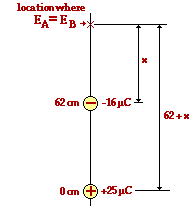A diagram is always a useful way of approaching the problem. Since A is positively charged, the electric field created by A is directed away from it. Since B is negatively-charged, the electric field created by B is directed towards. Thus, some directional reasoning would lead to the conclusion that the location along the axis must be outside of the two charges. And since object B has the smaller charge and creates the smaller E field, the location will be closer to object B than to object A. That is, what object B lacks in terms of charge it must make up for in terms of distance in order to have an equal field to object A's. The results of this reasoning are shown in the diagram at the right. The location of 0 electric field is a distance of x cm from the -16 µC charge and a distance of 62+x cm from the +25µC charge. These distances and charge values can be inserted into the equation above and algebra can be used to solve for x. (Note that the + and - sign has been dropped from the equations; directional meaning has been accounted for in the reasoning above.) k•(25 µC)/(62 + x)2 = k•(16 µC)/(x)2 Divide each side through by k. (25 µC)/(62 + x)2 = (16 µC)/(x)2 Take the square root of each side of the equation and then cross-multiply. 5/(62 + x) = 4/x 5x = 4•(62 + x) = 248 + 4x x = 248 Since x represents the distance from object B and since object B is at a y-coordinate of 62 cm, the answer to the problem is 310 cm.

 Useful Web Links Newton's Laws and the Electrical Force [ #33 | #34 | #35 | #36 | #37 | #38 | #39 | #40 | #41 | #42 | #43 ]

38. Three objects are located along the x-axis. Object A with a charge of +5.6 µC is located at the origin. Object B has a charge of -4.2 µC and is located at the -1.2 m location. Object C has a charge of +7.7 µC and is located at the +2.4 m location. Determine the magnitude and direction of the net electric force acting upon object A.

 Answer: 0.21 N, left Object A experiences two forces - the force of object B attracting it and pulling it leftward (FBA) and the force of object C repelling it and pushing it leftward (FCA). The diagram below depicts the situation and the forces.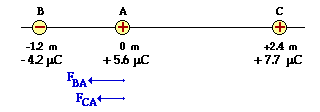The net electric force upon object A is simply the vector sum of these two forces. The strategy involves computing each force (FBA and FCA ) individually and then adding them together as vectors. The calculations of the two forces are shown below. (Note that the + and - signs have been dropped from the values on the charge since the directional information has already been accounted for in the analysis above.) FBA = k•QA•QB/d2 = (9.0x109 N/m2/C2)•(5.6x10-6 C)•(4.2 x10-6 C)/(1.2 m)2 = 0.147 N, left FCA = k•QA•QC/d2 = (9.0x109 N/m2/C2)•(5.6x10-6 C)•(7.7 x10-6 C)/(2.4 m)2 = 0.067 N, left Now the net electric force on object A can be determined by adding the two forces resulting from the interactions with A and with B. The net force is 0.21 N, left. (0.214 N rounded to two significant digits)

 Useful Web Links Newton's Laws and the Electrical Force [ #33 | #34 | #35 | #36 | #37 | #38 | #39 | #40 | #41 | #42 | #43 ]

39. Object A has a x-y coordinate position of (+5.0, 0.0). Object B has a x-y coordinate location of (0.0, +4.0). If object A has a charge of -5.8 µC and object B has a charge of +8.9 µC, then what is the resultant electric field strength at the origin.

 Answer: 5.4 x103 N/C, 293 degrees counter-clockwise from east Both charged object A and charged object B create an electric field, the strength of which is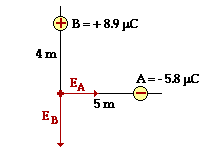dependent upon the distance from the charged object. This problem involves computing the electric field strength as created by both charges individually and as experienced at the origin (EA and EB). Since electric field strength is a vector, it can be added using rules of vector addition. An electric field vector always has a direction associated with the direction which a + test charge would be forced. Since object A is a negatively charged vector, the vector EA is directed towards it (i.e., rightwards). Since object B is a positively charged vector, the vector EB is directed away from it (i.e., downwards). Once computed individually, EA and EB will be added. The diagram at the right depicts the situation. The calculations below represent the mathematics. EA = kQA/RA2 = (9.0x109 N/m2/C2)•(5.8x10-6 C)/(5.0 m)2 = 2.088 x 103 N/C EB = kQB/RB2 = (9.0x109 N/m2/C2)•(8.9x10-6 C)/(4.0 m)2 = 5.006 x 103 N/C Now the two E values will be added as vectors. The sketch at the right depicts the addition.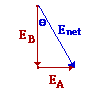Since they are at right angles to each other, the Pythagorean theorem will be used to determine the magnitude and trigonometry will be used to determine the direction. Enet = SQRT[(2.088 x 103 N/C)2 + (5.006 x 103 N/C)2] Enet = SQRT[2.942 x 106 N2/C2] = 5.4 x103 N/C Theta = invtan(EA/EB) = invtan[(2.088 x 103 N/C)/(5.006 x 103 N/C)] = 22.6 deg. So the direction of the vector is ~23 degrees to the right of down (east of south) or simply 293 degrees counter-clockwise from east.

 Useful Web Links Electric Field Intensity [ #33 | #34 | #35 | #36 | #37 | #38 | #39 | #40 | #41 | #42 | #43 ]

40. For the situation described in Question #39, what would be the magnitude of the net electric force upon a +1.3 µC charge if placed at the origin.

 Answer: 0.0070 N This is simply a matter of using the definition of electric field - the electric field is the force per coulomb of charge. Expressed mathematically, E = F/q and rearranged, the force is given by the equation F = E•q By substitution, F = (5.4 x103 N/C)•(1.3x10-6 C) = 0.0070 N

 Useful Web Links Electric Field Intensity [ #33 | #34 | #35 | #36 | #37 | #38 | #39 | #40 | #41 | #42 | #43 ]

41. Alpha particles (i.e., Helium nucleus) have a molar mass of 4.002 g/mol and consist of two protons and two neutrons.

1. Determine the charge of one alpha particle in units of Coulombs and the mass of one alpha particle in units of kg.
2. Suppose that Ernie Rufferthord (not to be confused with the scientist of gold foil fame) wishes to suspend an alpha particle in midair by attracting it to a bundle of electrons held a distance of 1.00-m above the alpha particle. How many electrons would Ernie need in his bundle to accomplish such an amazing feat?

 Answer: approx. 141 electrons a. This involves a conversion using Avogadro's number and the molar mass: mass = 1 alpha particle • (1 mol/6.02 x 1023 alpha particles) • (4.002 g/1 mol) • (1 kg/1000 g) mass = 6.65 x 10-27 kg   b. Ernie wishes to use the force of electrical attraction between the alpha particle and the electron bundle to balance the downward pull of gravity upon the alpha particle. So Felect = Fgrav k • Qalpha particle • Qe- bundle/R2 = malpha particle • g The charge on the alpha particle can be determined from its composition - it contains 2 protons (and 2 neutral neutrons), each having a charge of 1.6x10-19 C. So the charge of an alpha particle is 3.2x10-19 C. The only unknown remaining is the charge on the electron bundle. Rearrangement of the equation yields the following expression for the charge on the electron bundle: Qe- bundle = malpha particle • g • R2 / (k • Qalpha particle) Substitution of known values into this equation yields 2.26x10-17 C as the charge on the electron bundle. With each electron contributed 1.6x10-19 C per electron, there must be approximately 141 electrons in the bundle.

 Useful Web Links Newton's Laws and the Electrical Force [ #33 | #34 | #35 | #36 | #37 | #38 | #39 | #40 | #41 | #42 | #43 ]

42. A 1.19-gram charged balloon hangs from a 1.99-m string which is attached to the ceiling. A Van de Graaff generator is located directly below the location where the string attaches to the ceiling and is at the same height as the balloon. The string is deflected at an angle of 32.0 degrees from the vertical due to the presence of the electric field. Determine the charge on the Van de Graaff generator if the charge on the balloon is 2.27x10-12 C.

 Answer: 0.397 C The balloon in this problem is experiencing an electric force resulting from the interaction with the Van de Graaff generator. Diagram A illustrates the situation. The amount of charge on the Van de Graaff generator will be related to the distance of separation (R), the electrical force of repulsion, and the charge on the balloon (which is given). So the problem-solving strategy involves determining the electrical force of repulsion using a force analysis and then using Coulomb's law to determine the charge on the Van de Graaff generator.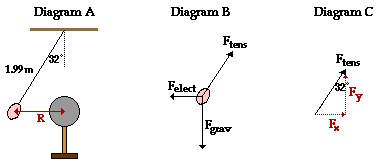Diagram B above shows a free-body diagram for the balloon. The three forces must balance since the balloon is at rest. Therefore, the x-component of the tension force (Fx in Diagram C) must equal the electrical force (Felect). And similarly, the y-component of the tension force (Fy in Diagram C) must equal the gravity force (Fgrav). A trigonometric function relating the angle of 32.0 degrees to these components of forces can be written. tan(32.0 deg) = opposite/adjacent = Fx /Fy And due to the equivalency between the components and the other two forces, it can also be stated that: tan(32.0 deg) = Felect / Fgrav The equation can be rearranged to solve for Felect; the expression for Fgrav and the related values can be substituted. Felect = Fgrav • tan(32.0 deg) = m•g•tan(32.0 deg) = (0.00119 kg)•(9.8 m/s/s)•tan(32.0 deg) Felect = 7.29 x 10-3 N Now the separation distance (R) between the balloon and the generator can be determined. Observe in Diagram A that the distance R is related to the length of the string and the angle with the vertical. Using the sine function, R can be determined as follows: sin(32.0 deg) = opposite/hypotenuse = R/1.99 m R = 1.99 m • sin(32.0 deg) = 1.05 m Now with Felect, R, and Q1 known, Q2 (the charge on the Van de Graaff generator) can be determined. Felect = k•Q1•Q2/R2 By rearranging, the equation becomes Q2 = Felect • R2 / (k•Q1) = (7.29 x 10-3 N) • (1.05)2 / [ (9.0x109 N•m2/C2) • (2.27x10-12 C)] Q2 = 0.397 C

 Useful Web Links Newton's Laws and the Electrical Force [ #33 | #34 | #35 | #36 | #37 | #38 | #39 | #40 | #41 | #42 | #43 ]

43. Ignoring trace elements, a typical elemental composition (by mass) of the human body is as follows:

 10.5 % H 66.3 % O 19.9 % C 3.3 % N
1. Use these percentages, the molar mass values, Avogadro's number, and the atomic numbers to determine the total number of electrons (and protons) in a 73-kg human body (160 pounds).
2. If these electrons and protons were placed 100 m apart (the distance of approximately one football field), then what would be the force of electrical attraction between them.

 Answer: See answers below. a. How many electrons are in your body? It's kind of a neat question; and remarkably, a student of Chemistry and Physics knows enough to come up with an estimate. The strategy involves first using the mass of the body, the percent mass values, the atomic mass values, and Avogadro's number to first determine the number of atoms of each element in the body; then use the atomic number of each element to determine the number of electrons contributed by each element (assuming each atom is neutral); finally add all the electrons to determine the total number of electrons. Here it goes: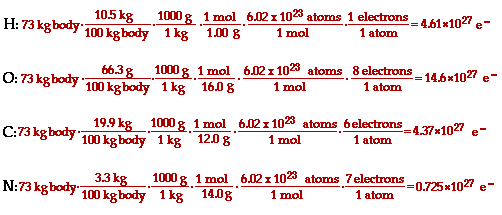When added, there are 24.3x1027 electrons (and a presumed equal number of protons).   b. The force of attraction of these protons and electrons at a separation distance of 100 m can easily be calculated if the net + and net - charge is known. Simply multiply the number of electrons by the charge of one electron (1.6x10-19 C); do similarly for the protons. The charge ends up being 3.9x109 C. Now Coulomb's law can be used to compute the electric force of attraction: Felect = k•Q1•Q2/R2 = (9.0x109 N•m2/C2)•(3.9x109 C)•(3.9x109 C)/(100 m)2 = 1.4x1025 N

 Useful Web Links Newton's Laws and the Electrical Force [ #33 | #34 | #35 | #36 | #37 | #38 | #39 | #40 | #41 | #42 | #43 ]

#### Navigate to:

Review Session Home - Topic Listing

Static Electricity - Home || Printable Version || Questions with Links

Answers to Questions:  All || #1-16 || #17-32 || #33-43

## You Might Also Like ...

Users of The Review Session are often looking for learning resources that provide them with practice and review opportunities that include built-in feedback and instruction. If that is what you're looking for, then you might also like the following:

The Calculator Pad includes physics word problems organized by topic. Each problem is accompanied by a pop-up answer and an audio file that explains the details of how to approach and solve the problem. It's a perfect resource for those wishing to improve their problem-solving skills.

2. Minds On Physics the App Series

Minds On Physics the App ("MOP the App") is a series of interactive questioning modules for the student that is serious about improving their conceptual understanding of physics. Each module of the series covers a different topic and is further broken down into sub-topics. A "MOP experience" will provide a learner with challenging questions, feedback, and question-specific help in the context of a game-like environment. It is available for phones, tablets, Chromebooks, and Macintosh computers. It's a perfect resource for those wishing to refine their conceptual reasoning abilities. Part 4 of the series includes topics in Static Electricity.

Visit: MOP the App Home || MOP the App - Part 4